## 6.9Reynolds-averaged simulation

The computational cost of DNS and, to a lesser extent, LES is too great for most practical CFD, as discussed in Sec. 6.8 . Instead, a Reynolds-averaged simulation (RAS) provides a much more aﬀordable method to calculate turbulence.

It solves equations for “averaged” ﬁeld variables to avoid resolving small ﬂuctuations. Rather than consider an average over a time interval, we imagine the same ﬂow repeated several times under nominally the same initial conditions (2 examples below).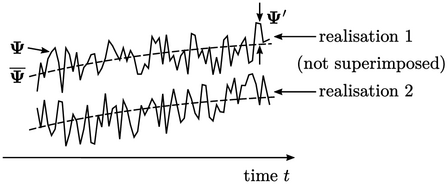Solutions vary due of diﬀerences in initial conditions and the chaotic nature of turbulence. The ensemble average calculates the mean solution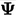for multiple realisations of the same ﬂow.

Each ﬁeld, e.g., is decomposed into the averaged ﬁeldand ﬁeld of random ﬂuctuations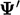, according to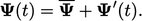(6.11)
We can apply this decomposition to ﬁelds in the momentum conservation Eq. (2.27 ) with stress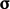split into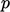and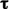as follows: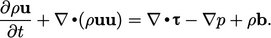(6.12)
Let us assume constant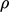and that the body force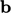is not subject to turbulent ﬂuctuations. Splitting the remaining ﬁelds, i.e.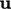,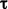and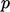, into instantaneous and ﬂuctuating components according to Eq. (6.11 ), and taking the ensemble average of Eq. (6.12 ), yields the following: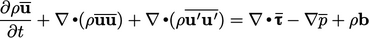(6.13)
The averaged Eq. (6.13 ) is derived using the following relations for general ﬁelds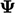and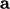: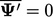;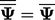;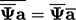;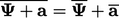. Relations for averaged derivatives are: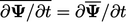;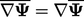;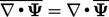.

### Reynolds stress

The terms for mean quantities in Eq. (6.13 ) are the same as in Eq. (6.12 ). The diﬀerence is that Eq. (6.13 ) includes the additional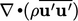term containing ﬂuctuations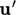.

The argument of this divergence derivative is a tensor known as the Reynolds stress11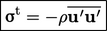(6.14)
Substituting Eq. (6.14 ) in Eq. (6.13) eliminates ﬂuctuation terms. The remaining equation is in terms of averaged properties only, so we can dispose of the average notation () to give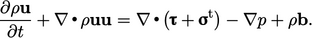(6.15)
This ensemble-averaged equation is the same form as Eq. (6.13 ) but with the addition of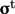. Solving this Reynolds-averaged equation is the key to low cost CFD with turbulence — but it requires a model for the additional unknown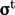.
11Osborne Reynolds, On the dynamical theory of incompressible viscous ﬂuids and the determination of the criterion, 1895.

Notes on CFD: General Principles - 6.9 Reynolds-averaged simulation